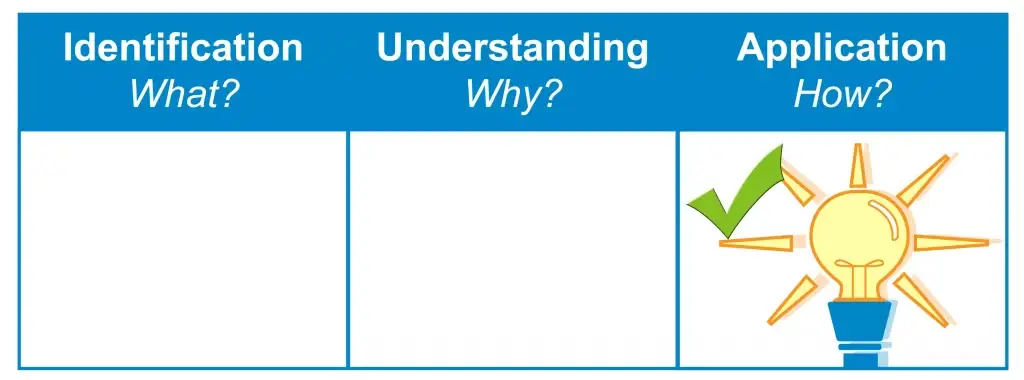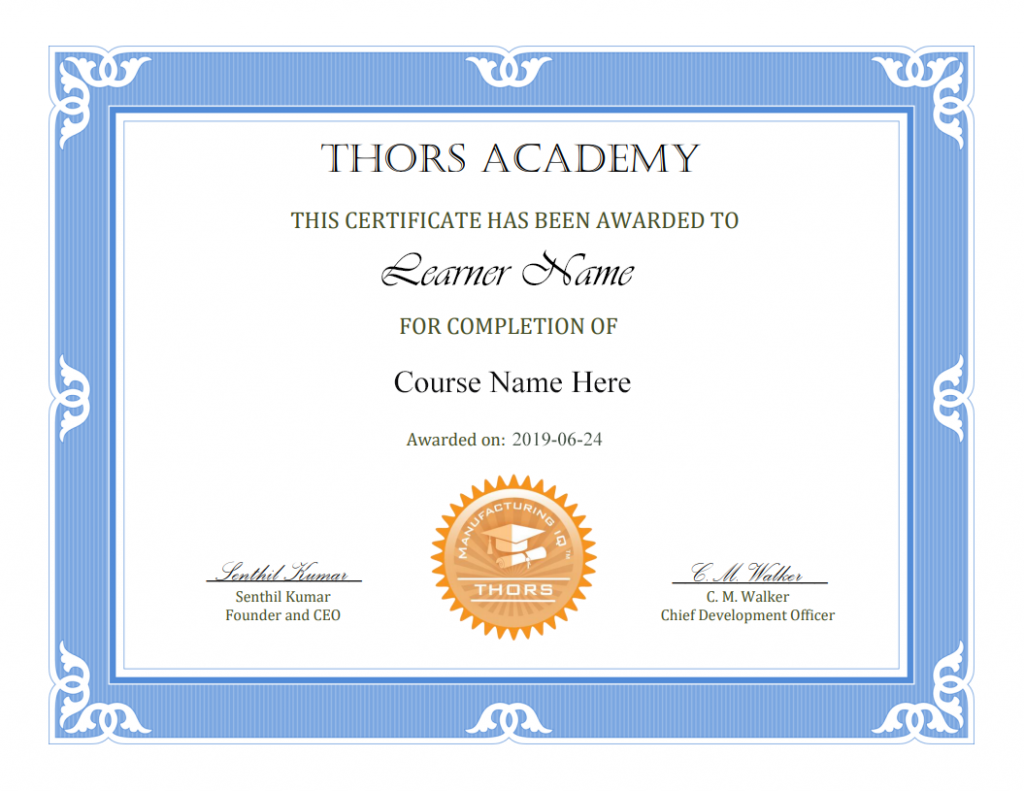THORS eLearning Solutions# Measurements: Measurement Math

\$180.00

The Measurements: Measurement Math course is an informative, entry-level introduction to the fundamental concepts required to better understand the measurement process.
Learning Hours: 2

## Course Description

The Measurements: Measurement Math course is an informative, entry-level introduction to the fundamental concepts required to better understand the measurement process. This course begins with an overview of the basic two-dimensional geometry of measurements, followed by an introduction to the common mathematical formulae that may be necessary for proper part inspection. Learners in the quality and manufacturing fields will find value in this course.

## Who will benefit from this Measurement Math course?

All Functions: Quality, Sales, Engineering, Manufacturing, Purchasing.

## Course Classification*THORS uses the Bloom’s Taxonomy Methodology for our course development.

## Certificate Awarded for Measurements: Measurement Math*upon successful completion

## Learning Objectives

1. Identify the two-dimensional geometry of measurements and their parts.
2. Calculate the various mathematical formulae that may be necessary during inspection.
3. Recognize how the two-dimensional geometry and measurement math apply to inspection.

1. Two-Dimensional Geometry of Measurements
1. Point
2. Line Segment
3. Plane
4. Circle
1. Degrees, Minutes, Seconds
1. Degree
2. Minute
3. Second
2. Diameter
4. Circumference
5. Arc
5. Angles
6. Triangles
7. Relationship of Features
1. Datums
2. Parallelism
3. Perpendicularity
4. Radius between Two Perpendicular Surfaces
2. Common Math Formulae
1. Circle Formulae
1. Circumference of a Circle
1. Calculating the Circumference of a Circle
2. Calculating Circumference Example 1
3. Calculating Circumference Example 2
2. Area of a Circle
1. Calculating the Area of a Circle
2. Calculating the Area of a Circle Example
2. Triangle Formulae
1. Area of a Triangle
1. Calculating the Area of a Triangle
2. Calculating the Area of a Triangle Example
2. The Pythagorean Theorem
1. The Pythagorean Theorem Formula
2. The Pythagorean Theorem Formula Example
3. Sine, Cosine, and Tangent
1. Calculating Sine, Cosine, and Tangent
2. Calculating Sine, Cosine, and Tangent Examples
3. Degrees, Minutes, Seconds Formulae
1. When Minutes and Seconds Add up to Less Than 60
2. When Minutes Add up to Greater Than or Equal to 60
3. When Seconds Add up to Greater Than or Equal to 60
4. When Minutes and Seconds Add up to Greater Than or Equal to 60
5. When Seconds Add up to Greater Than or Equal to 60 and the Final Answer Is a Complete Circle
2. Subtracting Degrees, Minutes, Seconds
1. When Subtraction Results in Enough Minutes and Seconds
2. When Subtraction Results in a Negative Amount of Seconds
3. When Subtraction Results in a Negative Amount of Minutes
4. When Subtraction Results in a Negative Amount of Minutes and Seconds
3. Converting DDD/MM/SS to Decimal Degrees
4. Converting Decimal Degrees to DDD/MM/SS